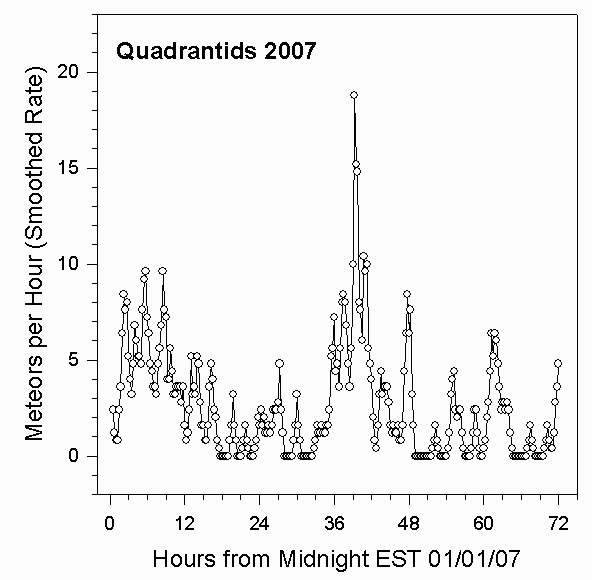Data Collection and Analysis

For details about our equipment and method of data analysis, see our main page. The 2007 Quadrantid meteor shower showed one major peak of nearly 20 meteors per hour at 3:15 PM EST on January 3, 2007, and considerable activity in the surrounding days. The peak occurred somewhat earlier than the prediction of 7:30 PM.

We've modified our data analysis. Instead of counting all meteors in an hour, we've counted meteors in quarter hour intervals, and then applied a data smoothing equation of the form

Smoothed_Rate(t) = 3.2*[((1/2)*N(t))+((1/4)*(N(t+1))+((1/4)*(N(t-1))+((1/8)*(N(t+2))+((1/8)*(N(t-2))]

where N(t) is the number of meteors in the fifteen minute interval t, N(t-1)is the number of meteors in the preceding fifteen minute interval, and so on.

The following graph shows the meteor count rates per hour we measured for the 2007 Quadrantids. These rates were determined by a computer program that counted all peaks that climbed at least 20% above the background level in one second. The graph shows the rates observed for the 72 hour period starting at Midnight EST on 01/01/07.Summary

We observed one peak for the 2007 Quadrantid meteor shower. The peak rate of about 19 meteors per hour occurred at 3:15 PM EST on January 3, 2007.